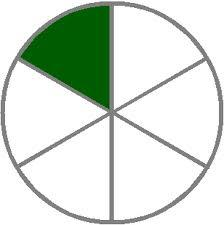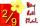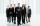# One sixth

How many sixths are two thirds?

x =  4

### Step-by-step explanation:Did you find an error or inaccuracy? Feel free to write us. Thank you!Tips to related online calculators
Need help to calculate sum, simplify or multiply fractions? Try our fraction calculator.
Check out our ratio calculator.

## Related math problems and questions:

• The ratioThe ratio of girls to boys in Mrs. White's class is 3:2.  If there are 12 boys, how many girls are in the class room?
• Report 2A School reports students to teacher ratio of 6:1. If there are 45 teachers in the School, how many students are there?
• Two thirdsFind two-thirds of the number equal to two-thirds of 99
• Division of moneyCalculate how many euros have Matthew, Miriam, Lucy, Michael, Janka when together have 2,700 euros and the amounts are at a ratio of 1:5:6:7:8.
• RatioWrite the ratio with other numbers so that the value is the same: 2: 9
• BarrelsPeter has 42 barrels. One-sixth of them are filled with lemonade, one third is filled with wine and half is empty. Calculate how many barrels are with each filling.
• Ratio6 numbers are in the ratio 1:5:1:5:5:5. Their sum is 242. What are the numbers?
• How many 16How many three-tenths are there in two and one-fourths?
• CompanyAfter increasing the number of employees by 15% company has 253 employees. How many employees take?
• Divide moneyDivide 1200 USD at a ratio of 1:2:3:4:5:6:9:10
• BuildingAt the building, we divided 240 boards into two piles in a 5: 3 ratio. How many were fewer boards in the lower pile?
• How manyHow many numbers are less than 222 with a digit sum is 8?
• PointsGryffindor won 437 points. How many points obtained by each of the faculties if they were split at a ratio of 5: 7: 3: 4?
• ExpressionIf it is true that (l + 15 w)/(w) = 6, then the value of the expression (13 l)/(12 w) is:
• AgesJohn, Teresa, Daniel and Paul have summary 56 years. Their ages are in a ratio of 1:2:5:6. Determine how many years have each of them.
• RatiosDivide: a) 250 CZK in the ratio 2:3 b) 1000 CZK in the ratio 4:7:9
• AndreAndre, Thomas, and Ivan split 88 postage stamps in a 2:5:4 ratio. How much did Thomas get?